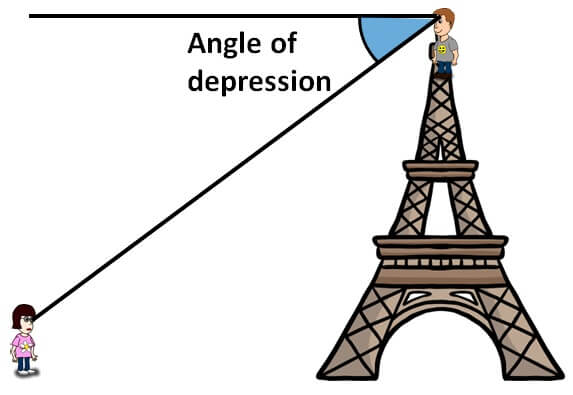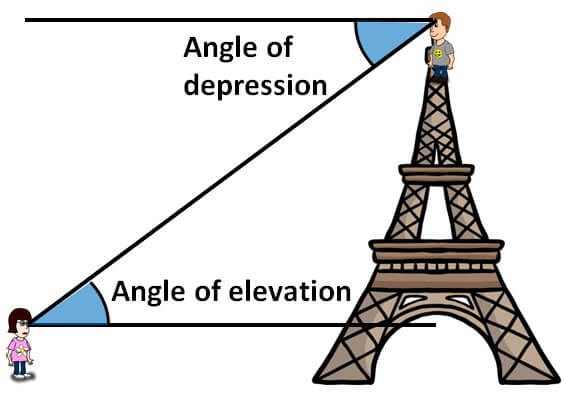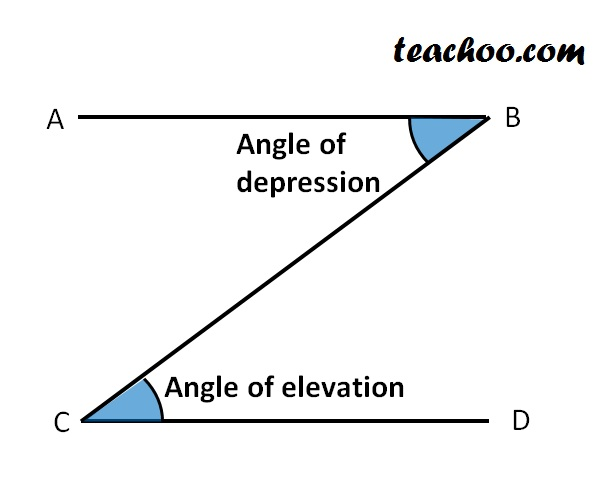Questions easy to difficult

Chapter 9 Class 10 Some Applications of Trigonometry
Concept wise
• Questions easy to difficult

In angle of depression, we studied thatHere, the angle of elevation of the girl to the boy isNow, the two horizontal lines are parallel,

and line of sight is the transversalAB and CD are parallel lines,

and BC is the transversal

∴ Angle of depression and angle of elevation are alternate angles, and they are equal

∴ Angle of depression = Angle of elevation

Thus, Angle of Depression from point A to point B is same as Angle of Elevation from point B to point A

Learn in your speed, with individual attention - Teachoo Maths 1-on-1 Class